# Non Verbal Reasoning - Classification

## Why Non Verbal Reasoning Classification?

In this section you can learn and practice Non Verbal Reasoning Questions based on "Classification" and improve your skills in order to face the interview, competitive examination and various entrance test (CAT, GATE, GRE, MAT, Bank Exam, Railway Exam etc.) with full confidence.

## Where can I get Non Verbal Reasoning Classification questions and answers with explanation?

IndiaBIX provides you lots of fully solved Non Verbal Reasoning (Classification) questions and answers with Explanation. Solved examples with detailed answer description, explanation are given and it would be easy to understand. All students, freshers can download Non Verbal Reasoning Classification quiz questions with answers as PDF files and eBooks.

## Where can I get Non Verbal Reasoning Classification Interview Questions and Answers (objective type, multiple choice)?

Here you can find objective type Non Verbal Reasoning Classification questions and answers for interview and entrance examination. Multiple choice and true or false type questions are also provided.

## How to solve Non Verbal Reasoning Classification problems?

You can easily solve all kind of Non Verbal Reasoning questions based on Classification by practicing the objective type exercises given below, also get shortcut methods to solve Non Verbal Reasoning Classification problems.

### Exercise :: Classification - Section 1

In each problem, out of the five figures marked (1), (2), (3), (4) and (5), four are similar in a certain manner. However, one figure is not like the other four. Choose the figure which is different from the rest.

1.

Choose the figure which is different from the rest.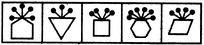(1)     (2)     (3)     (4)     (5)

 A. 1 B. 2 C. 3 D. 4 E. 5

Explanation:

The pins, equal in number to the number of sides in the main figure are attached to the midpoint of a side of the main figure in case of figures (2), (3), (4) and (5). In fig. (1), these pins are attached to a vertex of the main figure.

2.

Choose the figure which is different from the rest.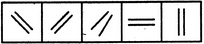(1)     (2)     (3)     (4)     (5)

 A. 1 B. 2 C. 3 D. 4 E. 5

Explanation:

In all other figures, the two line segments are parallel to each other.

3.

Choose the figure which is different from the rest.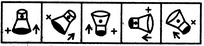(1)     (2)     (3)     (4)     (5)

 A. 1 B. 2 C. 3 D. 4 E. 5

Explanation:

In all other figures, the arrow and the V sign lie towards the black end of the main figure.

4.

Choose the figure which is different from the rest.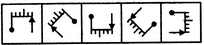(1)     (2)     (3)     (4)     (5)

 A. 1 B. 2 C. 3 D. 4 E. 5

Explanation:

In all other figures, there are two small line segments towards the pin and three small line segments towards the arrow.

5.

Choose the figure which is different from the rest.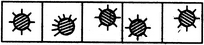(1)     (2)     (3)     (4)     (5)

 A. 1 B. 2 C. 3 D. 4 E. 5